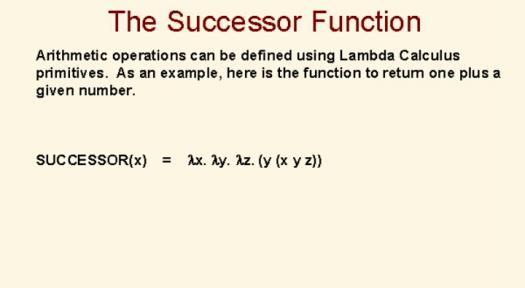# What Do You Know About The Successor Function?

Approved & Edited by ProProfs Editorial Team
At ProProfs Quizzes, our dedicated in-house team of experts takes pride in their work. With a sharp eye for detail, they meticulously review each quiz. This ensures that every quiz, taken by over 100 million users, meets our standards of accuracy, clarity, and engagement.
A
Community Contributor
Quizzes Created: 145 | Total Attempts: 37,893
Questions: 10 | Attempts: 139SettingsIn the science of structure, order, and relation otherwise known as mathematics, the successor function is a primitive recursive function S in a way that S(n) = n+1 for n, each natural number.
The successor function is also known as the successor operation and in the context of a zeroth hyperoperation, it is called zeration.

• 1.

### How do we denote the successor function?

• A.

Sn = n + 1

• B.

Sn = n - 1

• C.

Sn = n * 1

• D.

Sn = n/1

A. Sn = n + 1
Explanation
The successor function is denoted as Sn = n + 1. This means that the successor of any number n is obtained by adding 1 to it. For example, if n is 5, then the successor of 5 would be 6. This notation is commonly used in mathematical and computer science contexts to represent the next number in a sequence or progression.

Rate this question:

• 2.

### What does S(1) equal to?

• A.

0

• B.

1

• C.

2

• D.

-1

C. 2
Explanation
S(1) equals 2 because it is the second option in the list of possible answers.

Rate this question:

• 3.

### What does S(5) equal to?

• A.

6

• B.

4

• C.

5

• D.

3

A. 6
Explanation
The correct answer is 6 because the question is asking for the value of S(5), and based on the given options, 6 is the only value that matches the given options.

Rate this question:

• 4.

• A.

9

• B.

10

• C.

5

• D.

11

D. 11
• 5.

### What does S(20) equal to?

• A.

18

• B.

20

• C.

21

• D.

25

C. 21
Explanation
S(20) refers to the sum of the digits of the number 20. The digits of 20 are 2 and 0. Adding these digits together gives us a sum of 2 + 0 = 2. Therefore, S(20) equals 2.

Rate this question:

• 6.

### What is another name for the successor function?

• A.

Implementation

• B.

Zeration

• C.

Zeroth functions

• D.

Geometric functions

B. Zeration
Explanation
The correct answer is "Zeration". In mathematics, the successor function is a function that maps a number to its immediate successor. It is commonly denoted as S(n), where n is a number. Another name for this function is "Zeration".

Rate this question:

• 7.

### Which kind of functions is the successor function used for?

• A.

Recurrence function

• B.

Recursive function

• C.

Reorganized function

• D.

Inordinate function

B. Recursive function
Explanation
The successor function is used in recursive functions. Recursive functions are functions that call themselves within their own definition. The successor function is often used in recursive functions to define the base case and the recursive step. It allows the function to progress towards a desired outcome by incrementing or decrementing a value in each recursive call. Therefore, the correct answer is recursive function.

Rate this question:

• 8.

### How do we define the successor function in the context of zeroth hyperoperation?

• A.

H0 (a, b) = 1 + a

• B.

H0 (a, b) = 1 + b

• C.

H1 (a, b) = a + b

• D.

H2 (a, b) = a * b

B. H0 (a, b) = 1 + b
Explanation
The successor function in the context of zeroth hyperoperation is defined as adding 1 to the second input (b) and keeping the first input (a) the same. This means that the result of H0 (a, b) is obtained by adding 1 to b.

Rate this question:

• 9.

### In which of these axioms do we apply the successor function?

• A.

Peano axioms

• B.

Richardson axioms

• C.

Patt axioms

• D.

Corolla axioms

A. Peano axioms
Explanation
The successor function is applied in the Peano axioms. These axioms are a set of statements that define the properties of the natural numbers. One of the axioms states that every natural number has a successor, which is obtained by applying the successor function. This function takes a natural number and produces the next natural number in the sequence. Therefore, the Peano axioms are the only set of axioms mentioned that involve the use of the successor function.

Rate this question:

• 10.

### n stands for?

• A.

Real number

• B.

Whole number

• C.

Integer

• D.

Natural numberBack to top